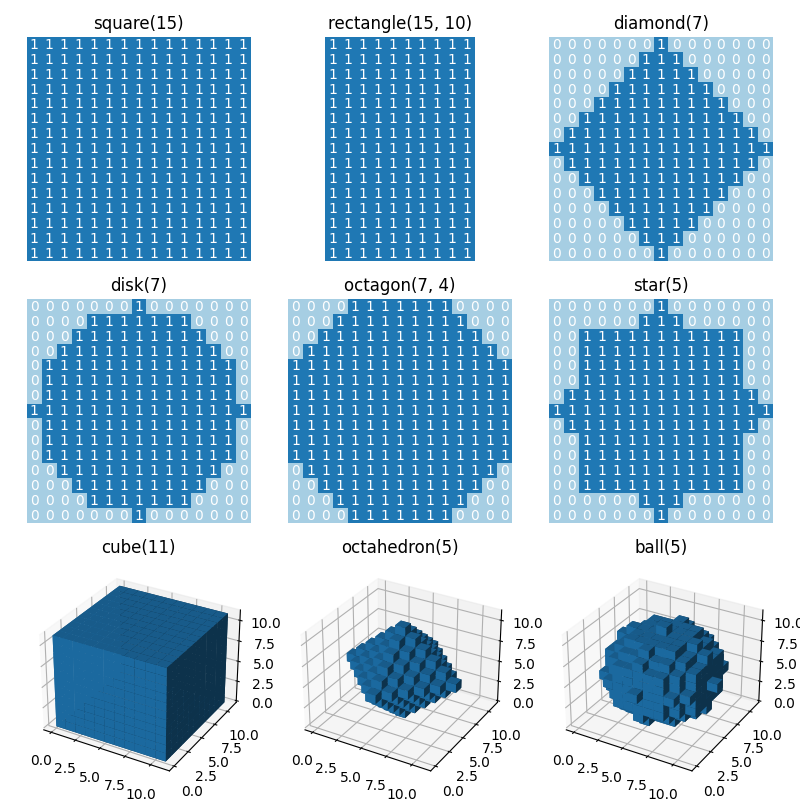# Generate footprints (structuring elements)¶

This example shows how to use functions in `skimage.morphology` to generate footprints (structuring elements) for use in morphology operations. The title of each plot indicates the call of the function.```import matplotlib.pyplot as plt
from mpl_toolkits.mplot3d import Axes3D

from skimage.morphology import (square, rectangle, diamond, disk, cube,
octahedron, ball, octagon, star)

# Generate 2D and 3D structuring elements.
struc_2d = {
"square(15)": square(15),
"rectangle(15, 10)": rectangle(15, 10),
"diamond(7)": diamond(7),
"disk(7)": disk(7),
"octagon(7, 4)": octagon(7, 4),
"star(5)": star(5)
}

struc_3d = {
"cube(11)": cube(11),
"octahedron(5)": octahedron(5),
"ball(5)": ball(5)
}

# Visualize the elements.
fig = plt.figure(figsize=(8, 8))

idx = 1
for title, struc in struc_2d.items():
ax.imshow(struc, cmap="Paired", vmin=0, vmax=12)
for i in range(struc.shape):
for j in range(struc.shape):
ax.text(j, i, struc[i, j], ha="center", va="center", color="w")
ax.set_axis_off()
ax.set_title(title)
idx += 1

for title, struc in struc_3d.items():
ax = fig.add_subplot(3, 3, idx, projection=Axes3D.name)
ax.voxels(struc)
ax.set_title(title)
idx += 1

fig.tight_layout()
plt.show()
```

Total running time of the script: ( 0 minutes 1.267 seconds)

Gallery generated by Sphinx-Gallery